### Time to event analysis (Survival analysis)

When modelling the time taken for an event to happen (e.g.death) we often use survival analysis rather than regression models. A common feature of these data is that for some subjects the event did not happen at all during the study period, called (right) censoring, and survival analysis can elegantly incorporate the information from these subjects. Regression models on the other hand have no easy way to include these subjects.

Survival analysis can often be applied to ecological data, some examples include

• Survival times for animals/plants

Stockwell, Michelle Pirrie, et al. “Effects of pond salinization on survival rate of amphibian hosts infected with the chytrid fungus.” Conservation Biology 29.2 (2015): 391-399.

• Germination timing

Donohue, Kathleen. “Germination timing influences natural selection on life-history characters in Arabidopsis thaliana.” Ecology 83.4 (2002): 1006-1016.

• Response to stimulus (drought)

Sperry, Jinelle H., and Patrick J. Weatherhead. “Prey-mediated effects of drought on condition and survival of a terrestrial snake.” Ecology 89.10 (2008): 2770-2776.

• Reproductive success

Mann, Janet, et al. “Female reproductive success in bottlenose dolphins (Tursiops sp.): life history, habitat, provisioning, and group-size effects.” Behavioral Ecology 11.2 (2000): 210-219.

#### Note: Survival analysis vs. analysis of survival data

It’s best to be careful when searching for “survival analysis”. Sometimes the term “survival analysis”" is used when analysing survival data using non-survival type methods which do not incorporate information from censoring, like regression.

### Censoring

#### Right censoring

If the time to event is only known to be greater than some amount then this is right censoring. This usually happens because the event of interest has not happen during the study period, or if a subject is lost to follow-up.

#### Left censoring

If the time to event is only known to be less than some amount then this is left censoring. This usually happens because the ‘exposure’ happens before the start of the study, but we don’t know when. For example we might know when the individual was diagnosed with a disease, but not how long before that they contracted it.

### Why not just use linear models?

Here is a quick simulated example of what happens.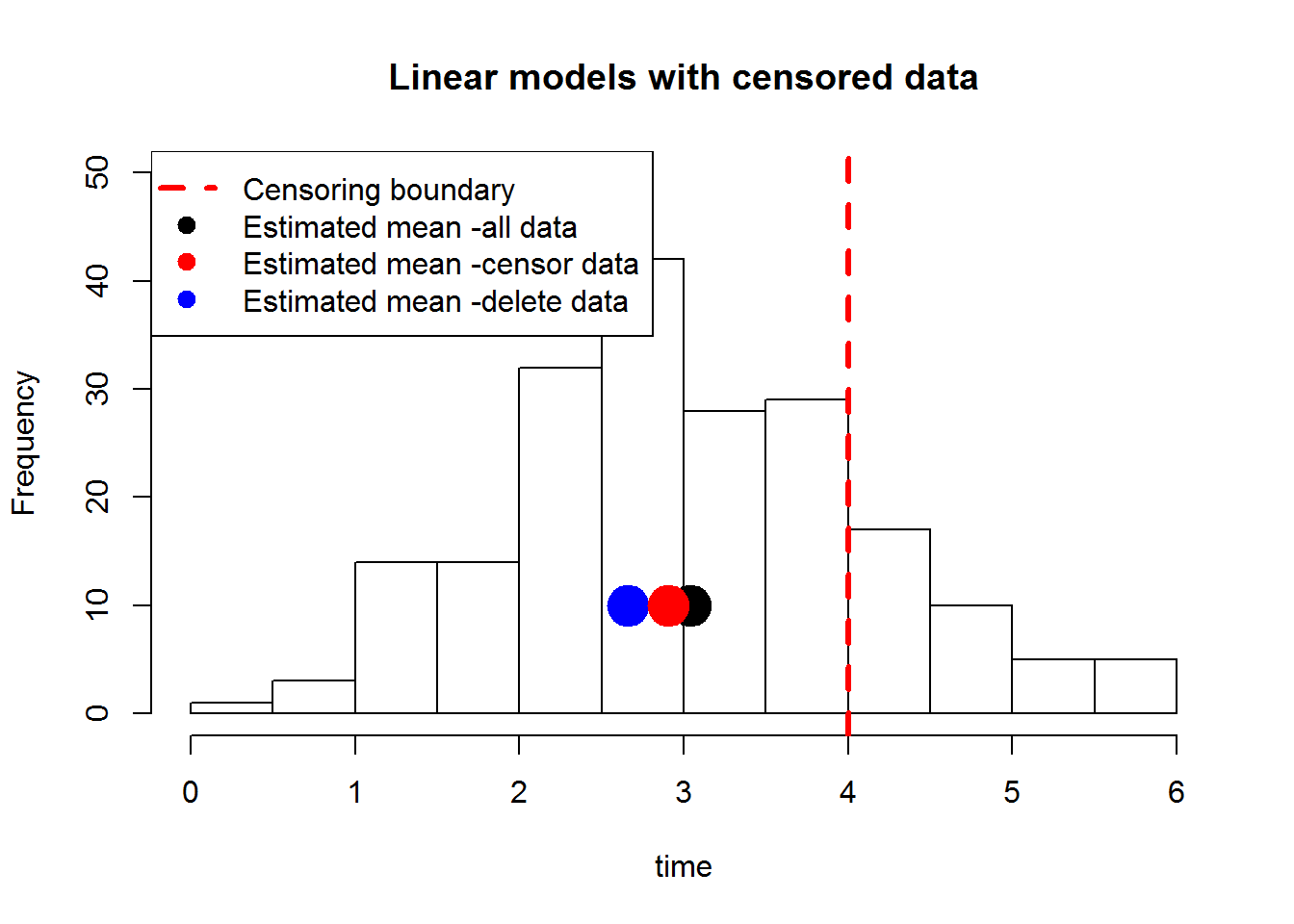I simulated some survival times with a mean of 3 hours. We will assume the experiment lasted for 4 hours, so some of the deaths are not observed, i.e. right censored. The true survival times are plotted, their mean is in the black dot. If we want to use linear models with time as the response we have to decide what to do with those individuals whose death we did not observe. We can set the times for all those individual that did not die by the end of the experiment to 4 (the end time for the experiment). If we do that and take the mean we get the red dot. If instead we leave those individuals out altogether and take the mean only of those subjects that die during the experiment we get the blue dot. Either way we are underestimating the true mean survival time.

### Fitting models

We have data of the time until flowers were visited by an insect, as well as information about the sex of the flower, size and which of 5 fields it was observed in. The data is right censored, i.e. some of the flowers were not visited by insects during the study period. See http://www.jstor.org/stable/pdf/1938524.pdf for the original data.

We will start by formatting the data using the Surv() function. For right censored data we only need two variables, the time of the event or censoring, and an indicator for each value (1 for observed, 0 for censored). To enter left censored or interval censored data look at ?Surv

library(survival)
## Loading required package: splines
flowers=read.csv("flowers.csv")
plants <- Surv(flowers$time,flowers$censor)

#### Categorical predictor

We’ll start with a very simple model with no predictors. This model is non-parametric. It does not assume anything about the shape of the survival curve. The survival curve is simply estimated as the proportion of those at risk who ‘survive’ at each time where there is a ‘death’.

my.fit=survfit(plants ~ 1)
plot(my.fit)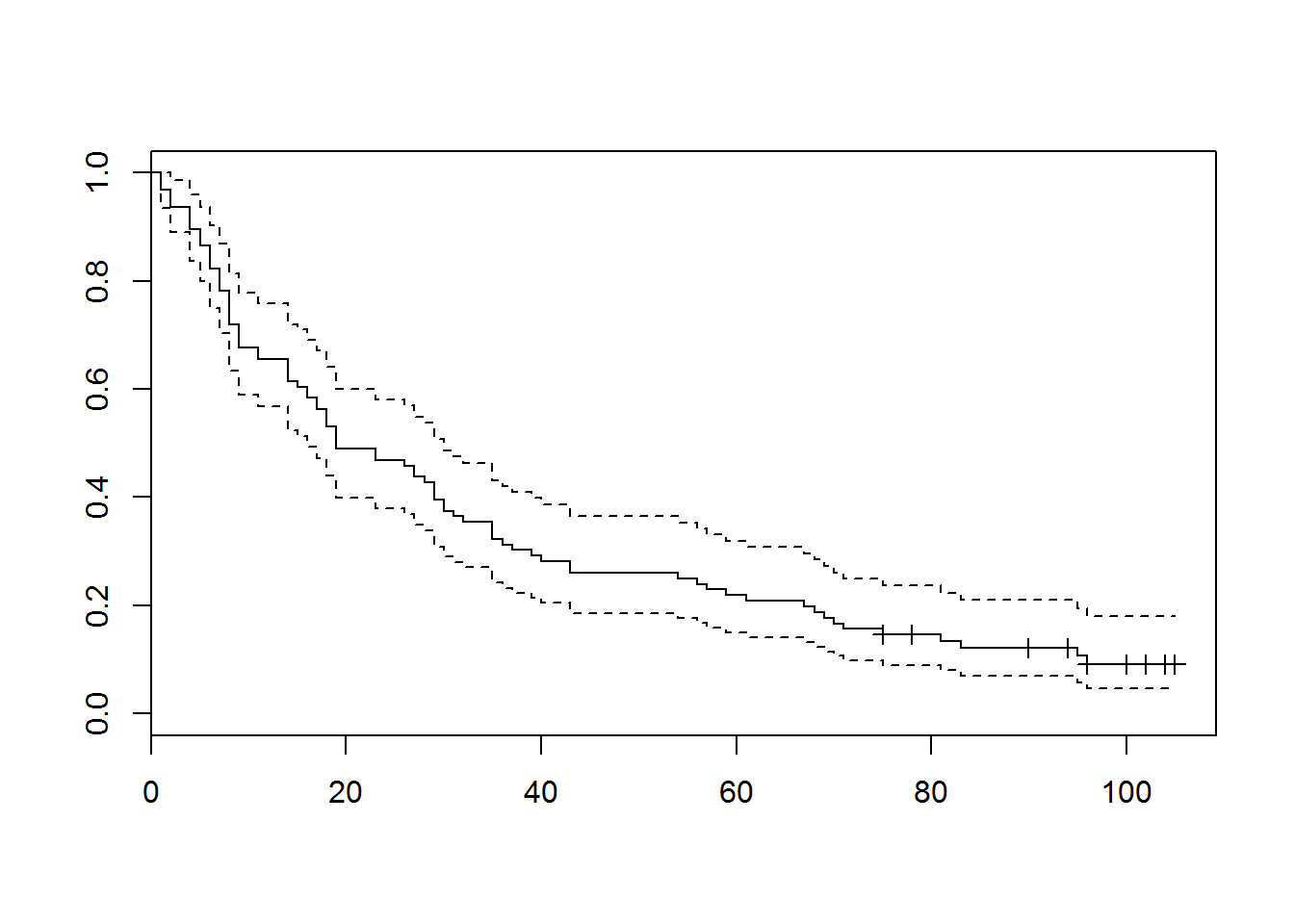This non-parametric model can be extended to include categorical predictors.

my.fit=survfit(plants ~ flowers$gender) plot(my.fit)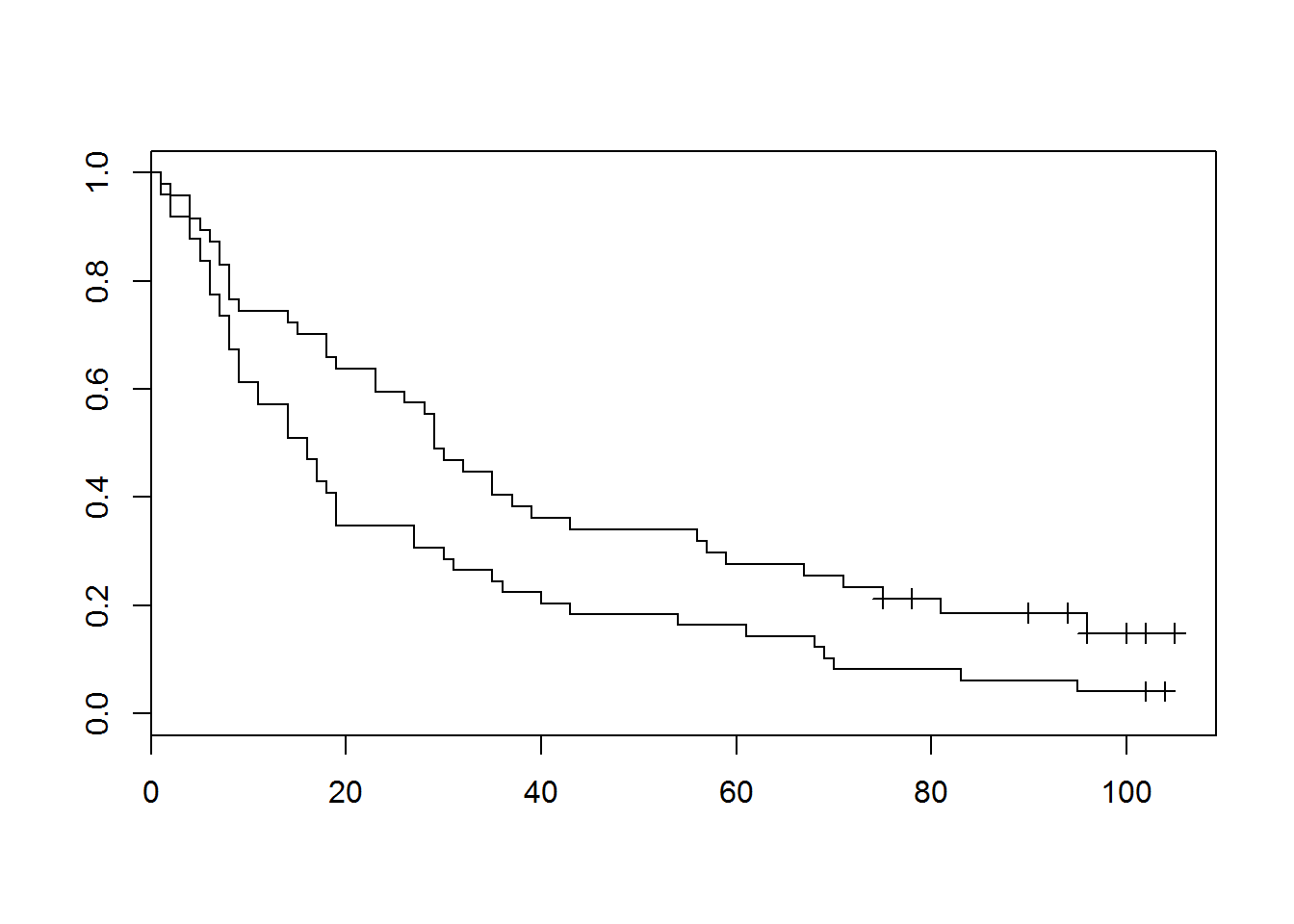my.diff <- survdiff( plants ~ flowers$gender )
my.diff 
## Call:
## survdiff(formula = plants ~ flowers$gender) ## ## N Observed Expected (O-E)^2/E (O-E)^2/V ## flowers$gender=female 47       39     49.9      2.39      5.96
## flowers$gender=male 49 47 36.1 3.30 5.96 ## ## Chisq= 6 on 1 degrees of freedom, p= 0.0146 The survdiff() function carries out a hypothesis test for our categorical variable and gives us a p-value of 0.0146. So there is some evidence of a gender difference in plants being visited by insects. #### Continuous predictors If we want to include continuous predictors the most common approach is a semi-parametric or fully parametric model. There are a few of these, the most commonly used is the Cox proportional hazard (CPH) model. This model assumes that the hazard (probability of death at each time given the subject is alive) is proportional for all subjects. So a covariate might act to multiply your hazard by a certain amount (e.g. being male doubles the flowers chance of being visited by an insect). To see the difference between this and the nonparametric model we will fit the same covariate. my.fit.cph=coxph(plants ~ gender,data=flowers) plot(survfit(my.fit.cph))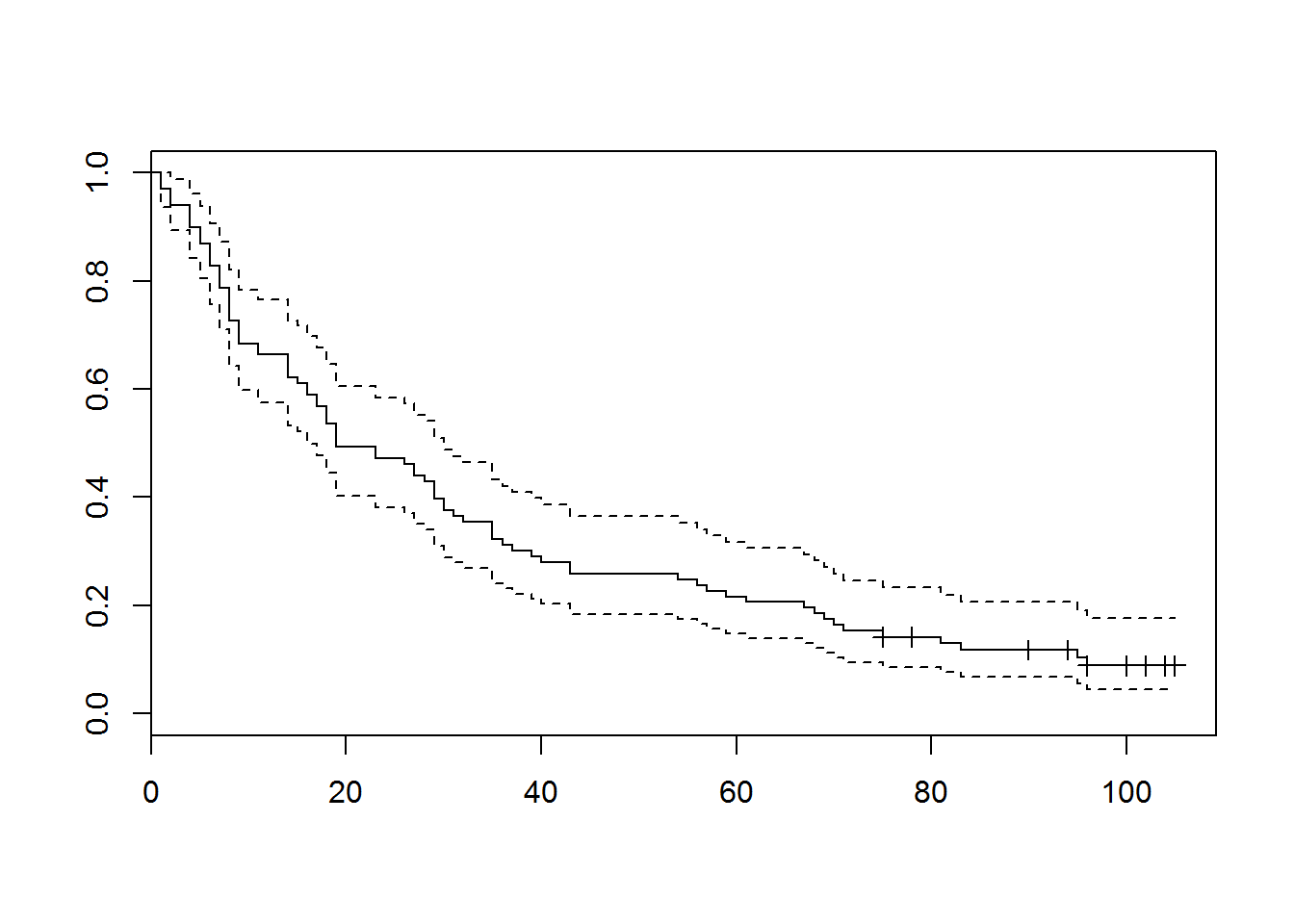newdat=data.frame(gender=levels(flowers$gender))
par(mfrow=c(1,2))
plot(survfit(my.fit.cph,newdata=newdat),col=1:2,main="CPH" )
plot(my.fit,main="Nonparametric")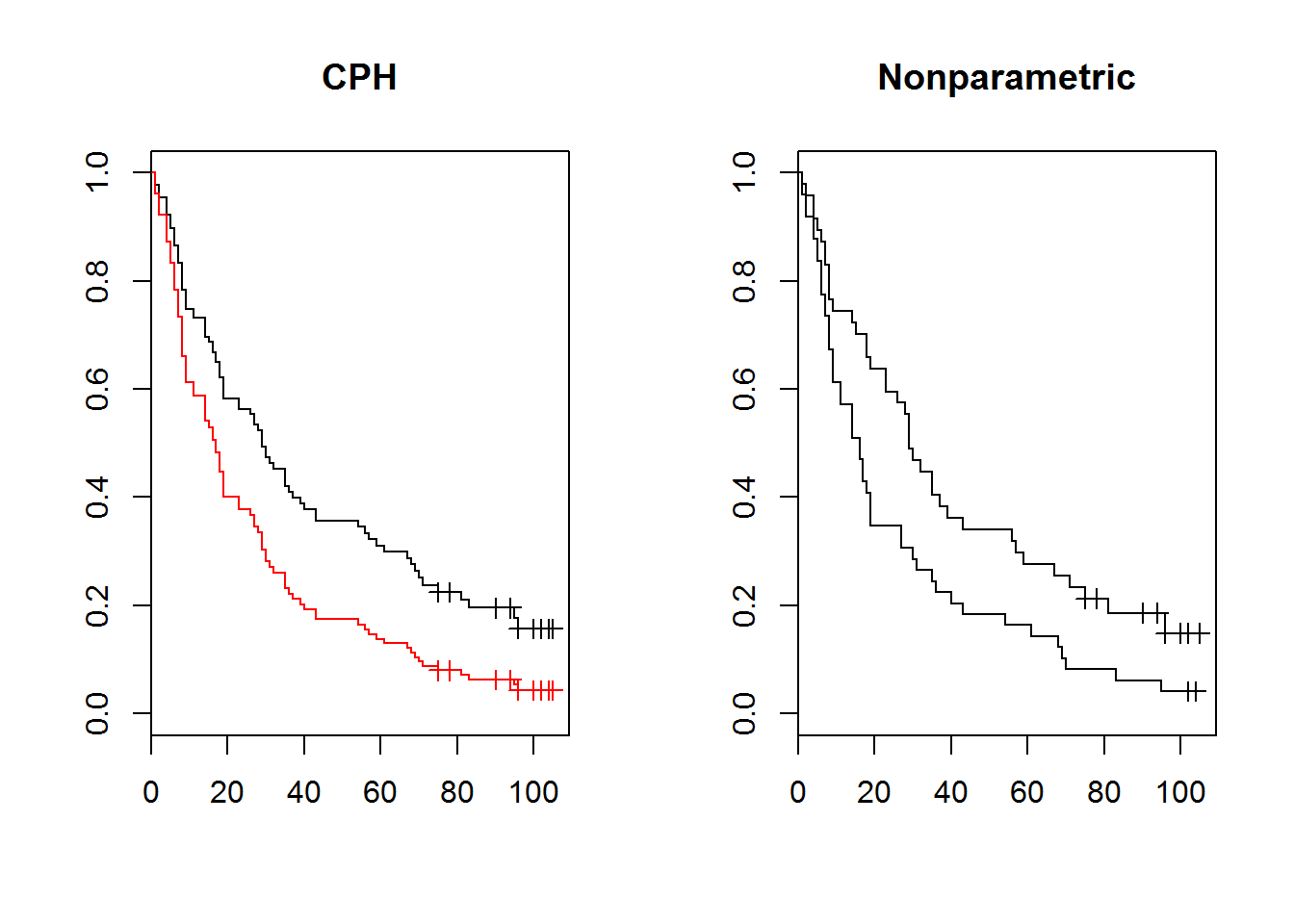If you look closely you can see the difference. Now let’s fit some continuous covariates and check assumptions.

my.fit.cph=coxph(plants ~ gender+size,data=flowers)
resid=residuals(my.fit.cph,"deviance")
plot(my.fit.cph$linear.predictor, resid)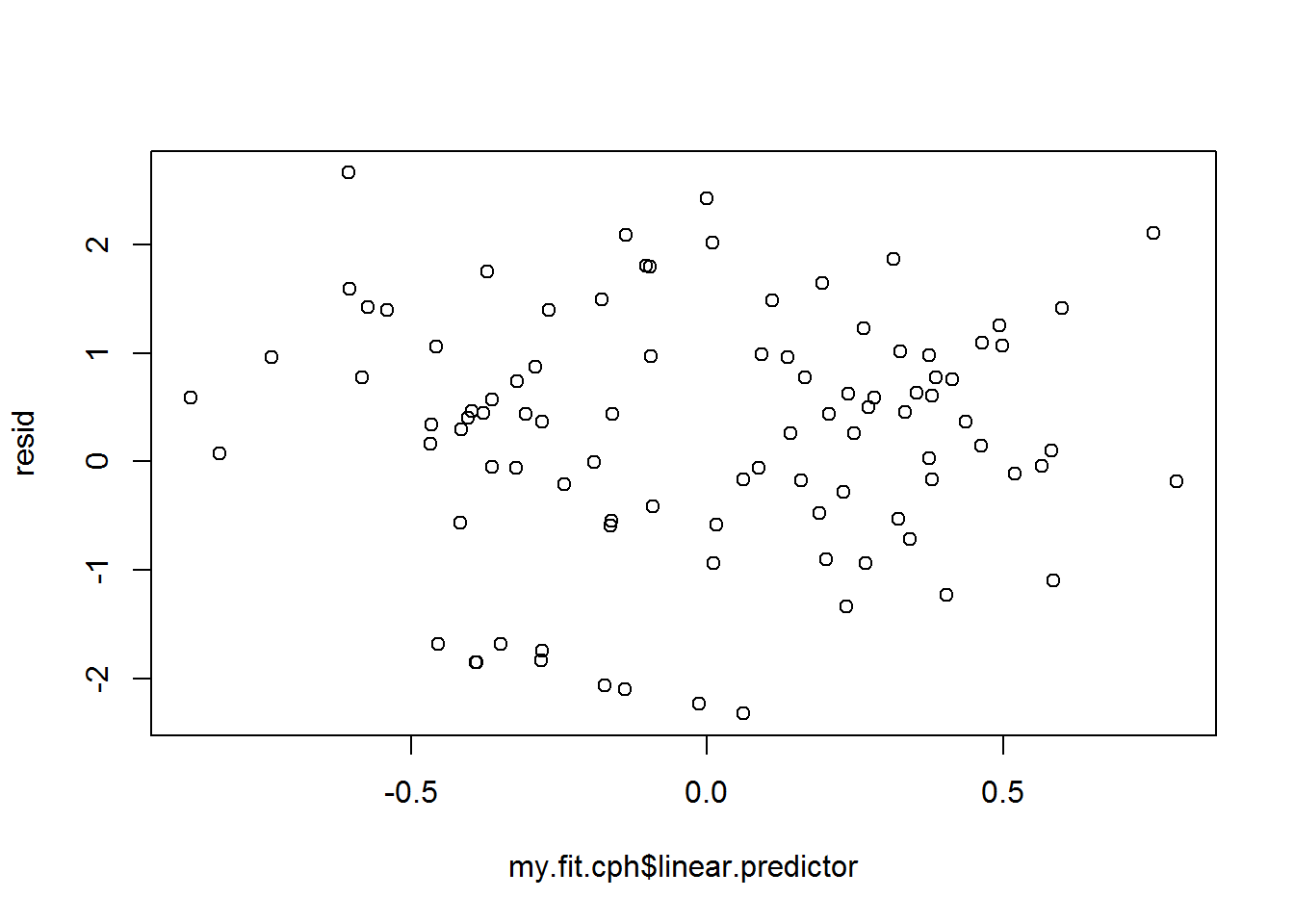A plot of deviance residuals can help you see violations of linearity in the predictors. The other assumption is the one about ‘proportional hazard’. To check this one we use the cox.zph() function. check=cox.zph(my.fit.cph) check ## rho chisq p ## gendermale 0.00196 0.00035 0.985 ## size -0.00588 0.00304 0.956 ## GLOBAL NA 0.00314 0.998 par(mfrow=c(1,2)) plot(check) abline(h=0,lty=3,col="red") plot(check) #there are two predictors abline(h=0,lty=3,col="red")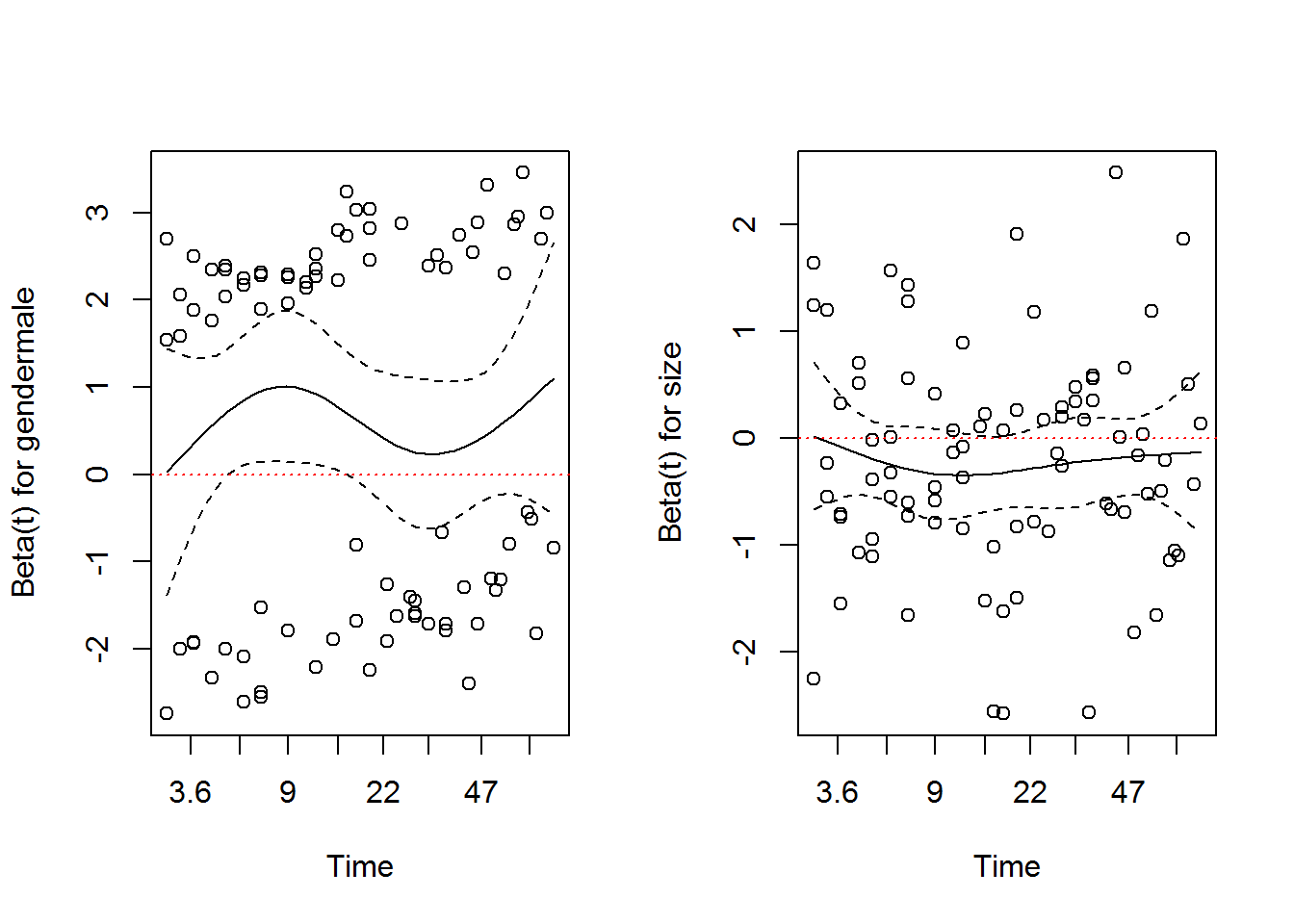In these plots we are looking for a trend in time, either increasing or decreasing. The p-values and plots both look reasonable. We can plot the model for various values of the parameters. newdat=expand.grid(gender=levels(flowers$gender),size=mean(flowers$size)) plot(survfit(my.fit.cph,newdata=newdat),col=1:2,main="CPH" )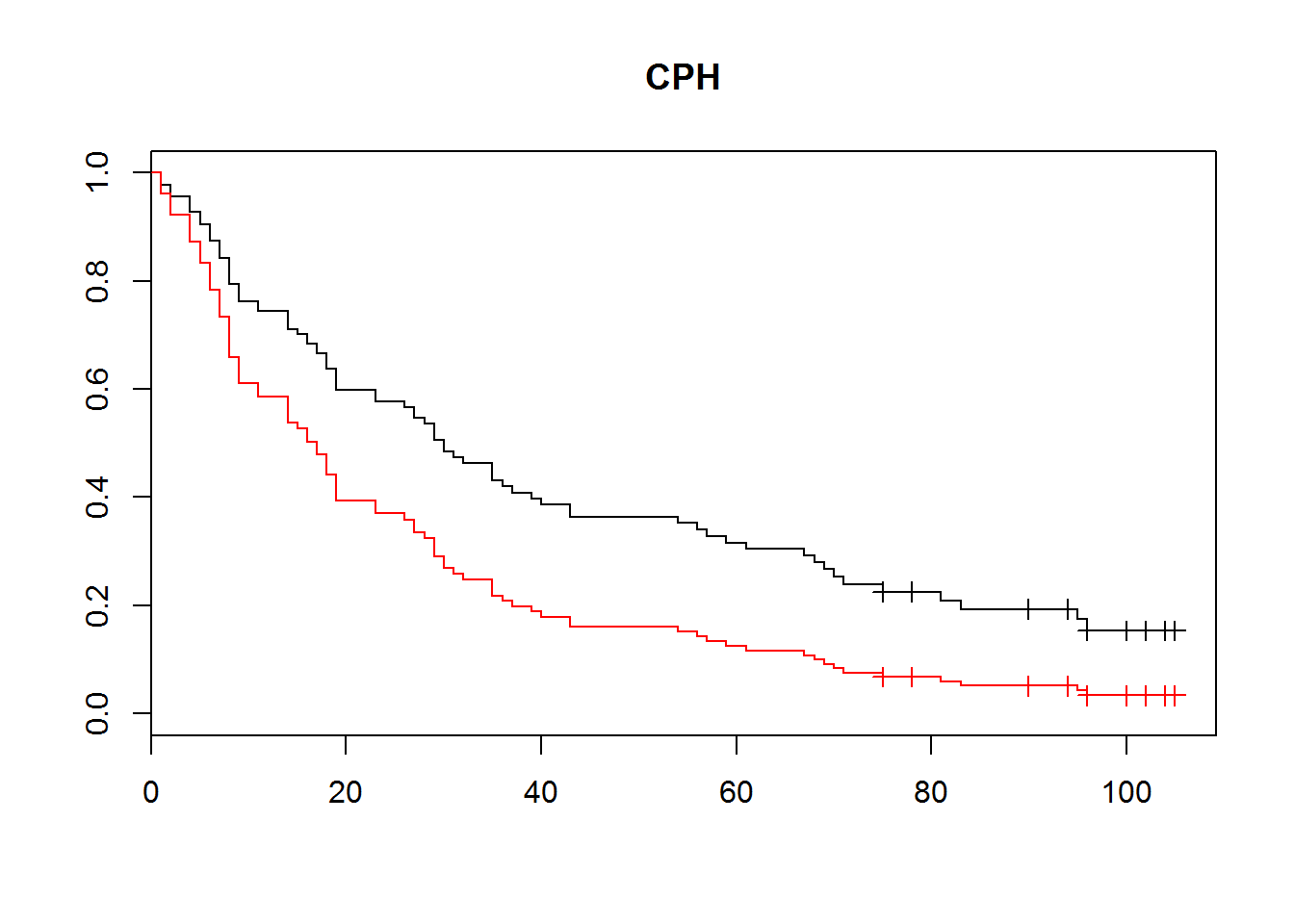This plots survival functions for each of the levels of gender, but always at the mean value of size. We can use the anova() and summary() functions for hypothesis testing. summary(my.fit.cph) ## Call: ## coxph(formula = plants ~ gender + size, data = flowers) ## ## n= 96, number of events= 86 ## ## coef exp(coef) se(coef) z Pr(>|z|) ## gendermale 0.5932 1.8097 0.2227 2.663 0.00774 ** ## size -0.2190 0.8033 0.1089 -2.012 0.04425 * ## --- ## Signif. codes: 0 '***' 0.001 '**' 0.01 '*' 0.05 '.' 0.1 ' ' 1 ## ## exp(coef) exp(-coef) lower .95 upper .95 ## gendermale 1.8097 0.5526 1.1695 2.8003 ## size 0.8033 1.2449 0.6489 0.9944 ## ## Concordance= 0.598 (se = 0.036 ) ## Rsquare= 0.098 (max possible= 0.999 ) ## Likelihood ratio test= 9.9 on 2 df, p=0.007074 ## Wald test = 9.66 on 2 df, p=0.007972 ## Score (logrank) test = 9.81 on 2 df, p=0.007403 my.fit.cph.null=coxph(plants ~ gender,data=flowers) anova(my.fit.cph.null,my.fit.cph) ## Analysis of Deviance Table ## Cox model: response is plants ## Model 1: ~ gender ## Model 2: ~ gender + size ## loglik Chisq Df P(>|Chi|) ## 1 -326.17 ## 2 -324.15 4.0517 1 0.04413 * ## --- ## Signif. codes: 0 '***' 0.001 '**' 0.01 '*' 0.05 '.' 0.1 ' ' 1 We have some evidence of an effect of size (p<0.05). We can also look at AIC’s of the models to do model selection. Unfortunately the AIC() function doesn’t work, so we have to extract the AIC from the model manually. c(extractAIC(my.fit.cph.null), extractAIC(my.fit.cph)) ##  654.3417 652.2900 ### Violation of proportional hazard assumption. If the proportional hazards assumption is violated we can stratify by the variable in question. This will fit separate nonparametric models for the different levels of the strata. The coefficients for the other predictors will still be shared. Our model seems to satisfy this assumption, but for illustration we’ll assume that gender violated the proportional hazard assumption, so we’ll stratify by gender. my.fit.cph.s=coxph(plants ~ strata(gender)+size,data=flowers) resid=residuals(my.fit.cph.s,"deviance") check=cox.zph(my.fit.cph.s) check ## rho chisq p ## size -0.00302 0.000812 0.977 par(mfrow=c(1,2)) plot(my.fit.cph.s$linear.predictor, resid)
plot(check)
abline(h=0,lty=3,col="red")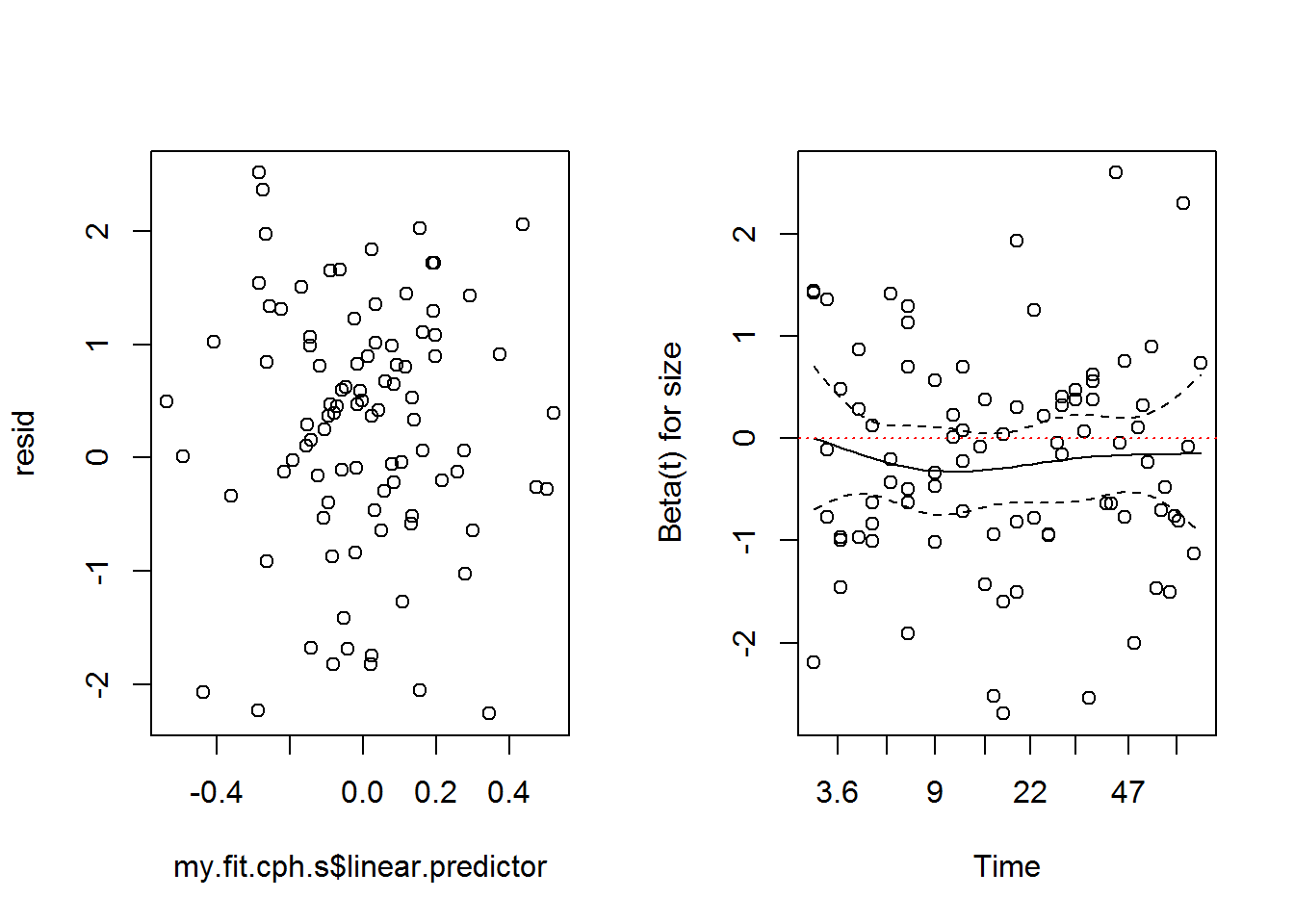summary(my.fit.cph.s)
## Call:
## coxph(formula = plants ~ strata(gender) + size, data = flowers)
##
##   n= 96, number of events= 86
##
##         coef exp(coef) se(coef)      z Pr(>|z|)
## size -0.2067    0.8132   0.1105 -1.871   0.0614 .
## ---
## Signif. codes:  0 '***' 0.001 '**' 0.01 '*' 0.05 '.' 0.1 ' ' 1
##
##      exp(coef) exp(-coef) lower .95 upper .95
## size    0.8132       1.23    0.6549      1.01
##
## Concordance= 0.55  (se = 0.052 )
## Rsquare= 0.036   (max possible= 0.996 )
## Likelihood ratio test= 3.5  on 1 df,   p=0.06124
## Wald test            = 3.5  on 1 df,   p=0.06136
## Score (logrank) test = 3.49  on 1 df,   p=0.06157

The p-value for size is now 0.06, which hasn’t changed that much, but has crossed the p=0.05 threshold. The downside of stratifying is that you now can’t examine the effect of the stratification variable, but you can plot it.

plot(survfit(my.fit.cph.s), col = 1:2)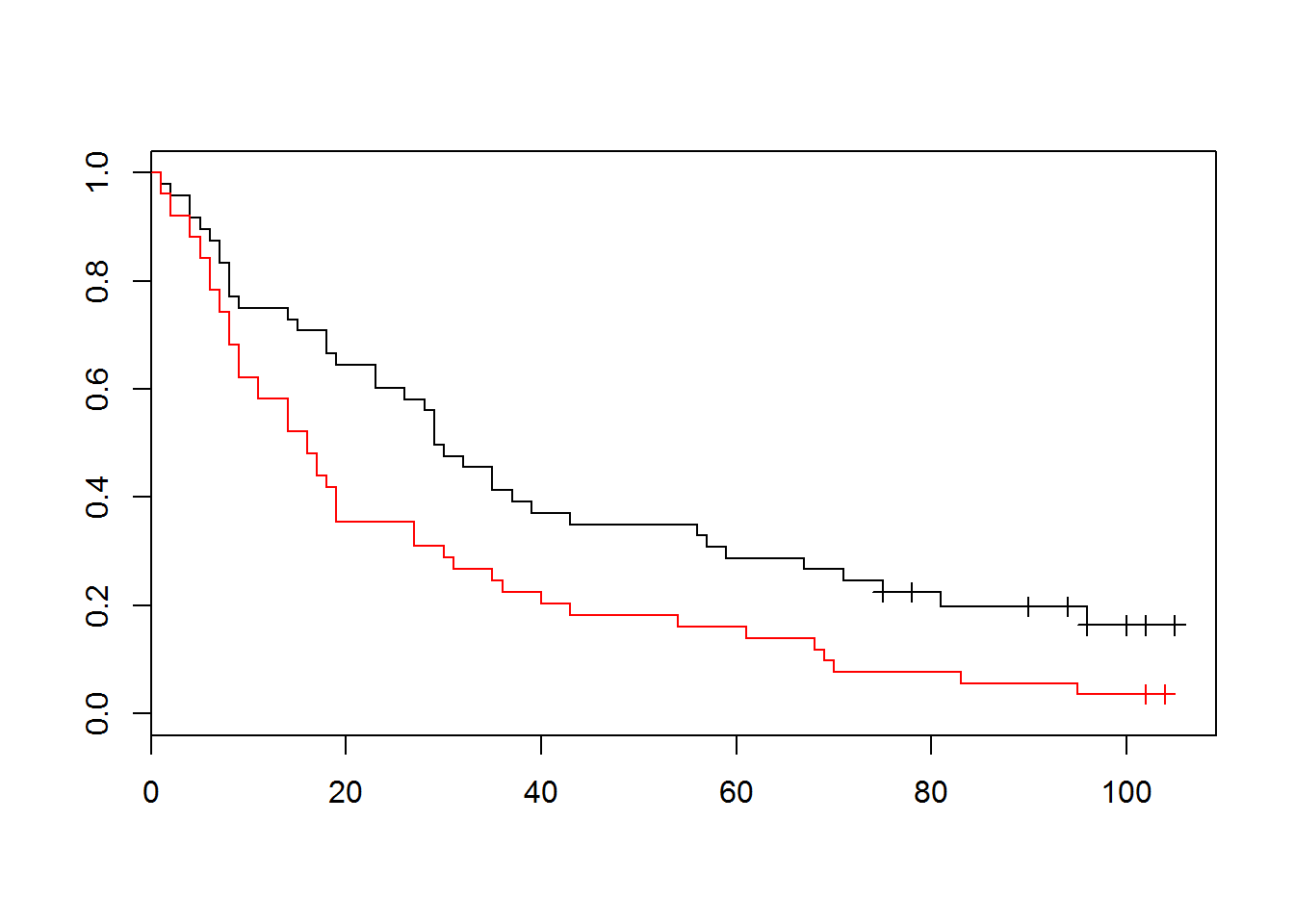### Mixed models

We can include random effects if we use the coxme package.

library(coxme)
## Loading required package: bdsmatrix
##
## Attaching package: 'bdsmatrix'
##
## The following object is masked from 'package:base':
##
##     backsolve
cph.mixed=coxme(plants ~ gender+size+(1|field),data=flowers)
anova(my.fit.cph,cph.mixed)
## Analysis of Deviance Table
##  Cox model: response is  plants
##  Model 1: ~gender + size
##  Model 2: ~gender + size + (1 | field)
##    loglik Chisq Df P(>|Chi|)
## 1 -324.15
## 2 -324.15 0.003  1    0.9561

Have a look at this for more on mixed models

https://cran.r-project.org/web/packages/coxme/vignettes/coxme.pdf

### Other things you might like to do

Time dependent covariates: https://cran.r-project.org/web/packages/survival/vignettes/timedep.pdf

Alternate parametric model : Accelerated failure time: Have a look at ?survreg

And much more: https://cran.r-project.org/web/views/Survival.html